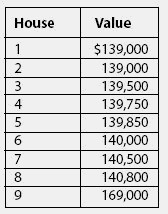# mean

(redirected from by any means)
Also found in: Dictionary, Thesaurus, Medical, Legal, Idioms, Encyclopedia.

## Mean

The expected value of a random variable. Arithmetic average of a sample.

## Arithmetic Mean Average

An average calculated by adding the value of the points in a data set and dividing the sum by the number of data points. For example, suppose one wishes to calculate the average income of a country with exactly five people in it, and their incomes are \$25,000, \$26,000, \$43,000, \$70,000, and \$72,000. It is calculated as:

(\$25,000 + \$26,000 + \$43,000 + \$70,000 + \$72,000) / 5 = \$47,200.

A limitation to the arithmetic mean average is that it can be overly affected by extremes in either direction. For example, if one of the five persons in the country earns \$100 billion per year, the arithmetic mean average income would be in the billions and would not accurately count the other four citizens. For this reason, many analysts use the median in conjunction with the arithmetic mean average. The arithmetic mean average is also called simply the mean.

## meanThe average of a set of numbers.Contrast with median,which is the middle figure in a set of numbers,and mode,which is the value that appears most often in a set of numbers.

Example: A survey of home values in a neighborhood of nine houses obtained the following values:

The mean, or average, is the total of all values divided by 9, or \$143,044.The median is the middle number when the numbers are all arranged from highest to lowest,which would be house 5, or \$139,850.The mode is \$139,000,because it is the number that appears most often.

Site: Follow: Share:
Open / Close